# Matplotlib.pyplot.streamplot() in Python

Stream plot is basically a type of 2D plot used majorly by physicists to show fluid flow and 2D field gradients .The basic function to create a stream plot in Matplotlib is:

`ax.streamplot(x_grid, y_grid, x_vec, y_vec, density=spacing)`

Here x_grid and y_grid are arrays of the x and y points.The x_vec and y_vec represent the stream velocity of each point present on the grid.The attribute #density=spacing# specify that how much close the streamlines are to be drawn together.

### Creating stream plot –

Let’s start by creating a simple stream plot that contains streamlines on a 10 by 10 grid.All the streamlines are parallel and pointing towards the right.The code below creates the stream plot containing horizontal parallel lines pointing to the right:

 `# Import libraries ` `import` `numpy as np ` `import` `matplotlib.pyplot as plt ` ` `  `# Creating dataset ` `x ``=` `np.arange(``0``, ``10``) ` `y ``=` `np.arange(``0``, ``10``) ` ` `  `# Creating grids ` `X, Y ``=` `np.meshgrid(x, y) ` ` `  `# x-component to the right ` `u ``=` `np.ones((``10``, ``10``))  ` ` `  `# y-component zero ` `v ``=` `np.zeros((``10``, ``10``))  ` ` `  `fig ``=` `plt.figure(figsize ``=` `(``12``, ``7``)) ` ` `  `# Plotting stream plot ` `plt.streamplot(X, Y, u, v, density ``=` `0.5``) ` ` `  `# show plot ` `plt.show() `

Output: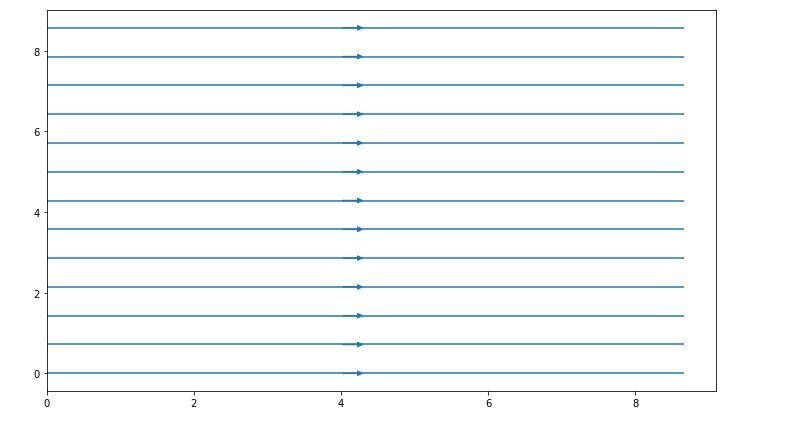Here, x and y are 1D arrays on an evenly spaced grid, u and v are 2D arrays of velocities of x and y where the number of rows should match with the length of y while the number of columns should match with x, density is a float value which controls the closeness of the stream lines.

### Customization of stream plot –

With the help of `streamplot()` function we can create and customize a plot showing field lines based on defined 2D vector field. Many attributes are available in `streamplot()` function for the modification of the plots.

 `# Import libraries ` `import` `numpy as np ` `import` `matplotlib.pyplot as plt ` ` `  `# Creating data set ` `w ``=` `3` `Y, X ``=` `np.mgrid[``-``w:w:``100j``, ``-``w:w:``100j``] ` `U ``=` `-``1` `-` `X``*``*``2` `+` `Y ` `V ``=` `1` `+` `X ``-` `Y``*``*``2` `speed ``=` `np.sqrt(U``*``*``2` `+` `V``*``*``2``) ` ` `  `# Creating plot ` `fig ``=` `plt.figure(figsize ``=` `(``12``, ``7``)) ` `plt.streamplot(X, Y, U, V, density ``=` `1``) ` ` `  `# show plot ` `plt.show() `

Output: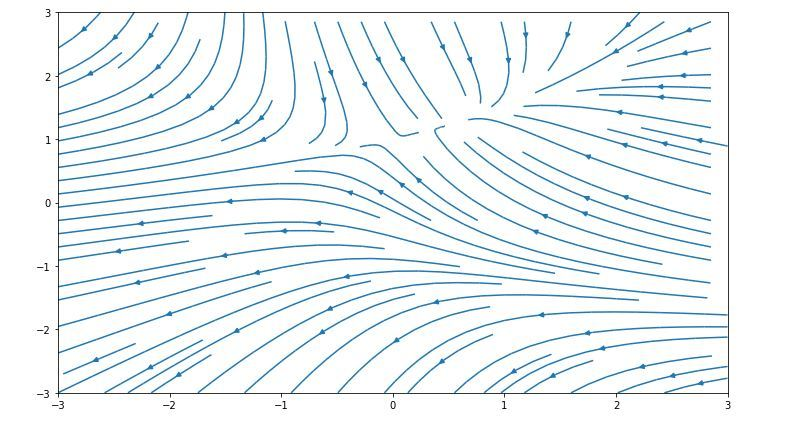Some of the customization of the above graph are listed below:

Varying the density of streamlines –

 `import` `numpy as np ` `import` `matplotlib.pyplot as plt ` `import` `matplotlib.gridspec as gridspec ` ` `  `# Creating dataset ` `w ``=` `3` `Y, X ``=` `np.mgrid[``-``w:w:``100j``, ``-``w:w:``100j``] ` `U ``=` `-``1` `-` `X``*``*``2` `+` `Y ` `V ``=` `1` `+` `X ``-` `Y``*``*``2` `speed ``=` `np.sqrt(U``*``*``2` `+` `V``*``*``2``) ` ` `  `fig ``=` `plt.figure(figsize ``=``(``24``, ``20``)) ` `gs ``=` `gridspec.GridSpec(nrows ``=` `3``, ncols ``=` `2``, ` `                       ``height_ratios ``=``[``1``, ``1``, ``2``]) ` ` `  `# Varying the density along a  ` `# streamline ` `ax ``=` `fig.add_subplot(gs[``0``, ``0``]) ` `ax.streamplot(X, Y, U, V,  ` `              ``density ``=``[``0.4``, ``0.8``]) ` ` `  `ax.set_title(``'Varying the density along a streamline'``) ` ` `  `# show plot ` `plt.tight_layout() ` `plt.show() `

Output: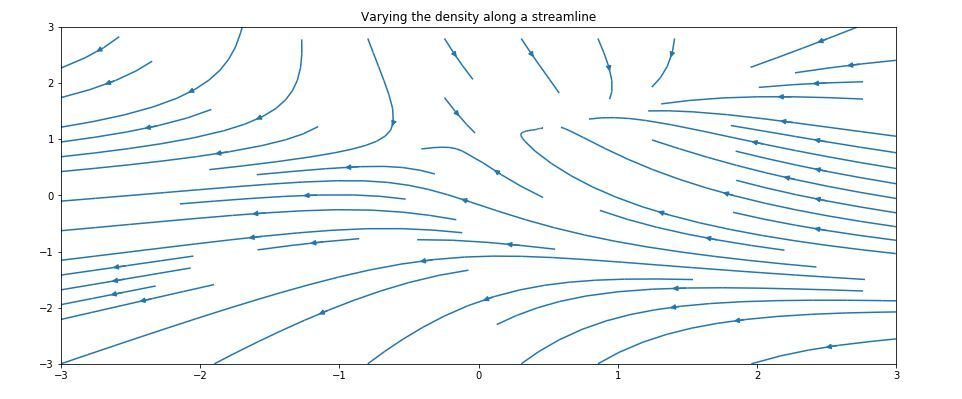Varying the color along a streamline –

 `import` `numpy as np ` `import` `matplotlib.pyplot as plt ` `import` `matplotlib.gridspec as gridspec ` ` `  `# Creating dataset ` `w ``=` `3` `Y, X ``=` `np.mgrid[``-``w:w:``100j``, ``-``w:w:``100j``] ` `U ``=` `-``1` `-` `X``*``*``2` `+` `Y ` `V ``=` `1` `+` `X ``-` `Y``*``*``2` `speed ``=` `np.sqrt(U``*``*``2` `+` `V``*``*``2``) ` ` `  `fig ``=` `plt.figure(figsize ``=``(``24``, ``20``)) ` `gs ``=` `gridspec.GridSpec(nrows ``=` `3``, ncols ``=` `2``, ` `                       ``height_ratios ``=``[``1``, ``1``, ``2``]) ` ` `  `# Varying color along a streamline ` `ax ``=` `fig.add_subplot(gs[``0``, ``1``]) ` `strm ``=` `ax.streamplot(X, Y, U, V, color ``=` `U, ` `                     ``linewidth ``=` `2``, cmap ``=``'autumn'``) ` `fig.colorbar(strm.lines) ` `ax.set_title(``'Varying the color along a streamline.'``) ` ` `  `# show plot ` `plt.tight_layout() ` `plt.show()     `

Output: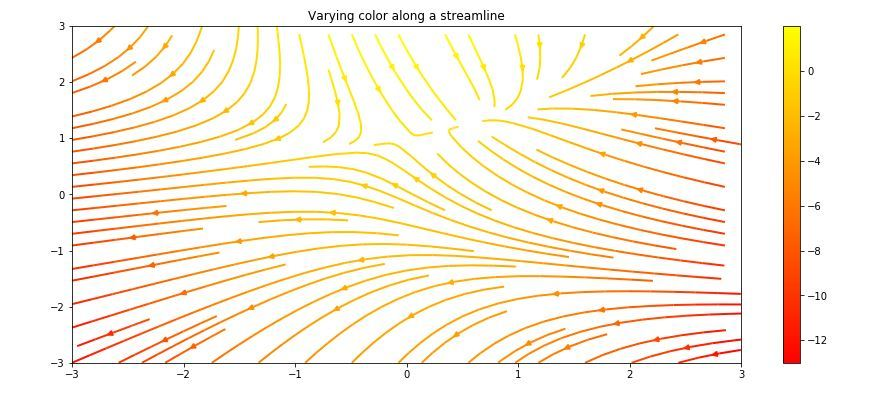Varying the line width along a streamline –

 `import` `numpy as np ` `import` `matplotlib.pyplot as plt ` `import` `matplotlib.gridspec as gridspec ` ` `  `# Creating dataset ` `w ``=` `3` `Y, X ``=` `np.mgrid[``-``w:w:``100j``, ``-``w:w:``100j``] ` `U ``=` `-``1` `-` `X``*``*``2` `+` `Y ` `V ``=` `1` `+` `X ``-` `Y``*``*``2` `speed ``=` `np.sqrt(U``*``*``2` `+` `V``*``*``2``) ` ` `  `fig ``=` `plt.figure(figsize ``=``(``24``, ``20``)) ` `gs ``=` `gridspec.GridSpec(nrows ``=` `3``, ncols ``=` `2``,  ` `                       ``height_ratios ``=``[``1``, ``1``, ``2``]) ` ` `  `# Varying line width along a streamline ` `ax ``=` `fig.add_subplot(gs[``1``, ``0``]) ` `lw ``=` `5` `*` `speed ``/` `speed.``max``() ` `ax.streamplot(X, Y, U, V, density ``=` `0.6``,  ` `              ``color ``=``'k'``, linewidth ``=` `lw) ` ` `  `ax.set_title(``'Varying line width along a streamline'``) ` ` `  `# show plot ` `plt.tight_layout() ` `plt.show() `

Output: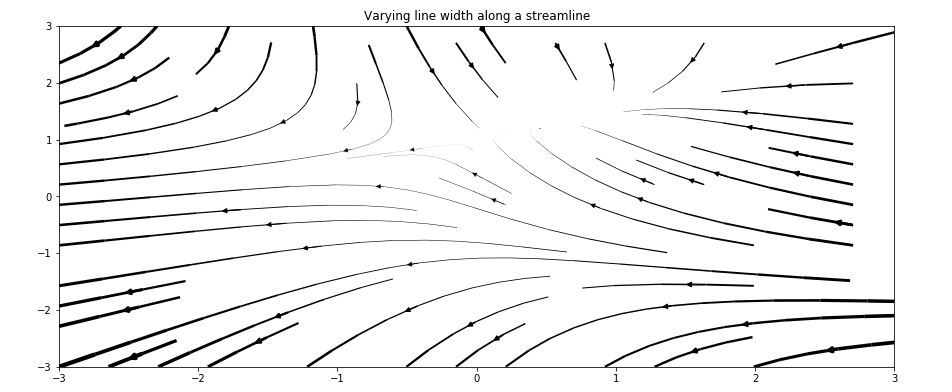Controlling the starting points of streamlines –

 `import` `numpy as np ` `import` `matplotlib.pyplot as plt ` `import` `matplotlib.gridspec as gridspec ` ` `  `# Creating dataset ` `w ``=` `3` `Y, X ``=` `np.mgrid[``-``w:w:``100j``, ``-``w:w:``100j``] ` `U ``=` `-``1` `-` `X``*``*``2` `+` `Y ` `V ``=` `1` `+` `X ``-` `Y``*``*``2` `speed ``=` `np.sqrt(U``*``*``2` `+` `V``*``*``2``) ` ` `  `fig ``=` `plt.figure(figsize ``=``(``24``, ``20``)) ` `gs ``=` `gridspec.GridSpec(nrows ``=` `3``, ncols ``=` `2``, ` `                       ``height_ratios ``=``[``1``, ``1``, ``2``]) ` ` `  `# Controlling the starting points ` `# of the streamlines ` `seek_points ``=` `np.array([[``-``2``, ``-``1``, ``0``, ``1``, ``2``, ``-``1``], ` `                        ``[``-``2``, ``-``1``,  ``0``, ``1``, ``2``, ``2``]]) ` ` `  `ax ``=` `fig.add_subplot(gs[``1``, ``1``]) ` `strm ``=` `ax.streamplot(X, Y, U, V, color ``=` `U,  ` `                     ``linewidth ``=` `2``, ` `                     ``cmap ``=``'autumn'``, ` `                     ``start_points ``=` `seek_points.T) ` ` `  `fig.colorbar(strm.lines) ` `ax.set_title('Controlling the starting\ ` `points of the streamlines') ` ` `  `# Displaying the starting points ` `# with blue symbols. ` `ax.plot(seek_points[``0``], seek_points[``1``], ``'bo'``) ` `ax.``set``(xlim ``=``(``-``w, w), ylim ``=``(``-``w, w)) ` ` `  `# show plot ` `plt.tight_layout() ` `plt.show() `

Output: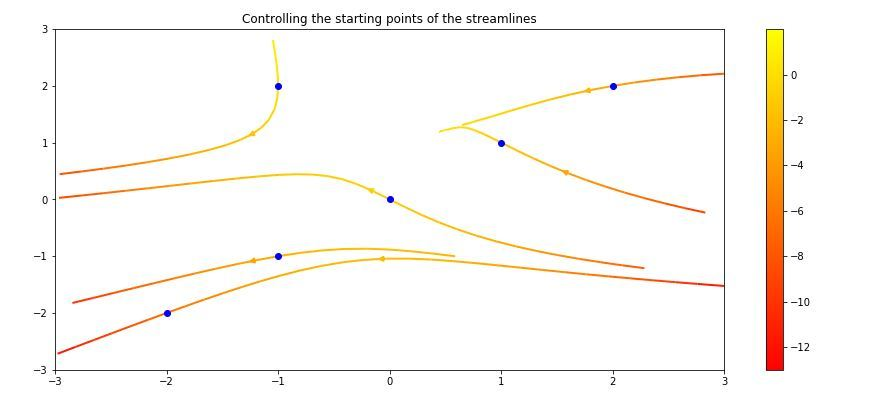Streamlines skipping masked regions and NaN values –

 `# Import libraries ` `import` `numpy as np ` `import` `matplotlib.pyplot as plt ` `import` `matplotlib.gridspec as gridspec ` ` `  `# Creating dataset ` `w ``=` `3` `Y, X ``=` `np.mgrid[``-``w:w:``100j``, ``-``w:w:``100j``] ` `U ``=` `-``1` `-` `X``*``*``2` `+` `Y ` `V ``=` `1` `+` `X ``-` `Y``*``*``2` `speed ``=` `np.sqrt(U``*``*``2` `+` `V``*``*``2``) ` ` `  `fig ``=` `plt.figure(figsize ``=``(``20``, ``16``)) ` `gs ``=` `gridspec.GridSpec(nrows ``=` `3``, ncols ``=` `2``, height_ratios ``=``[``1``, ``1``, ``2``]) ` ` `  `# Create a mask ` `mask ``=` `np.zeros(U.shape, dtype ``=` `bool``) ` `mask[``40``:``60``, ``40``:``60``] ``=` `True` `U[:``20``, :``20``] ``=` `np.nan ` `U ``=` `np.ma.array(U, mask ``=` `mask) ` ` `  `ax ``=` `fig.add_subplot(gs[``2``:, :]) ` `ax.streamplot(X, Y, U, V, color ``=``'r'``) ` `ax.set_title(``'Streamplot with Masking'``) ` ` `  `ax.imshow(~mask, extent ``=``(``-``w, w, ``-``w, w), alpha ``=` `0.5``, ` `          ``interpolation ``=``'nearest'``, cmap ``=``'gray'``, aspect ``=``'auto'``) ` `ax.set_aspect(``'equal'``) ` ` `  `# show plot ` `plt.tight_layout() ` `plt.show() `

Output: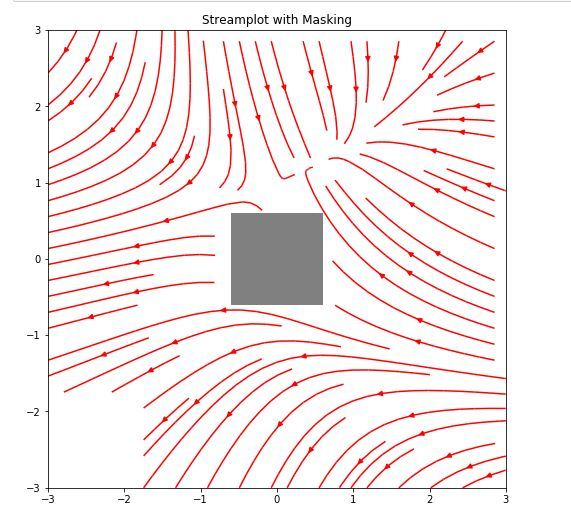Example:
Stream plot to demonstrate the electric field due to two point charges.The electric field at any point on a surface depends upon the position and distance between the two charges:

 `import` `sys ` `import` `numpy as np ` `import` `matplotlib.pyplot as plt ` `from` `matplotlib.patches ``import` `Circle ` ` `  `# Function to determin electric field ` `def` `E(q, r0, x, y): ` `    ``den ``=` `np.hypot(x``-``r0[``0``], y``-``r0[``1``])``*``*``3` `    ``return` `q ``*` `(x ``-` `r0[``0``]) ``/` `den, q ``*` `(y ``-` `r0[``1``]) ``/` `den ` ` `  `# Grid of x, y points ` `nx, ny ``=` `64``, ``64` `x ``=` `np.linspace(``-``2``, ``2``, nx) ` `y ``=` `np.linspace(``-``2``, ``2``, ny) ` `X, Y ``=` `np.meshgrid(x, y) ` ` `  `# Create a multipole with nq charges of ` `# alternating sign, equally spaced ` `# on the unit circle. ` ` `  `# Increase the power with increase in charge ` `nq ``=` `2``*``*``1`  `charges ``=` `[] ` `for` `i ``in` `range``(nq): ` `    ``q ``=` `i ``%` `2` `*` `2` `-` `1` `    ``charges.append((q, (np.cos(``2` `*` `np.pi ``*` `i ``/` `nq), ` `                        ``np.sin(``2` `*` `np.pi ``*` `i ``/` `nq)))) ` ` `  `# Electric field vector, E =(Ex, Ey) ` `# as separate components ` `Ex, Ey ``=` `np.zeros((ny, nx)), np.zeros((ny, nx)) ` ` `  `for` `charge ``in` `charges: ` `    ``ex, ey ``=` `E(``*``charge, x ``=` `X, y ``=` `Y) ` `    ``Ex ``+``=` `ex ` `    ``Ey ``+``=` `ey ` ` `  `fig ``=` `plt.figure(figsize ``=``(``18``, ``8``)) ` `ax ``=` `fig.add_subplot(``111``) ` ` `  `# Plotting the streamlines with  ` `# proper color and arrow ` `color ``=` `2` `*` `np.log(np.hypot(Ex, Ey)) ` `ax.streamplot(x, y, Ex, Ey, color ``=` `color, ` `              ``linewidth ``=` `1``, cmap ``=` `plt.cm.inferno, ` `              ``density ``=` `2``, arrowstyle ``=``'->'``,  ` `              ``arrowsize ``=` `1.5``) ` ` `  `# Add filled circles for the charges ` `# themselves ` `charge_colors ``=` `{``True``: ``'#AA0000'``,  ` `                 ``False``: ``'#0000AA'``} ` ` `  `for` `q, pos ``in` `charges: ` `    ``ax.add_artist(Circle(pos, ``0.05``,  ` `                         ``color ``=` `charge_colors[q>``0``])) ` ` `  `ax.set_xlabel(``'X-axis'``) ` `ax.set_ylabel(``'X-axis'``) ` `ax.set_xlim(``-``2``, ``2``) ` `ax.set_ylim(``-``2``, ``2``) ` `ax.set_aspect(``'equal'``) ` ` `  `plt.show() `

Output: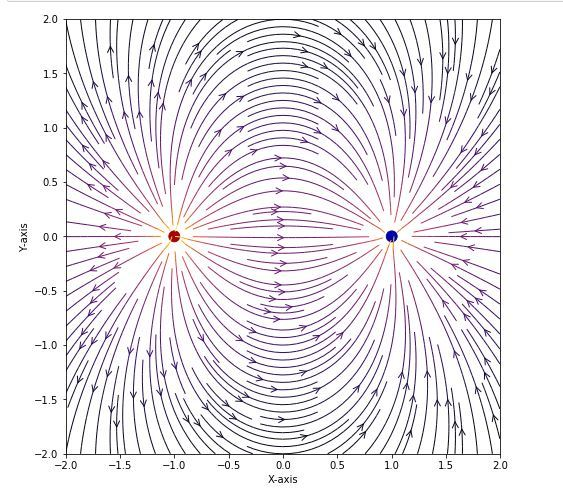Attention geek! Strengthen your foundations with the Python Programming Foundation Course and learn the basics.

To begin with, your interview preparations Enhance your Data Structures concepts with the Python DS Course.

My Personal Notes arrow_drop_upCheck out this Author's contributed articles.

If you like GeeksforGeeks and would like to contribute, you can also write an article using contribute.geeksforgeeks.org or mail your article to contribute@geeksforgeeks.org. See your article appearing on the GeeksforGeeks main page and help other Geeks.

Please Improve this article if you find anything incorrect by clicking on the "Improve Article" button below.

Article Tags :

Be the First to upvote.

Please write to us at contribute@geeksforgeeks.org to report any issue with the above content.